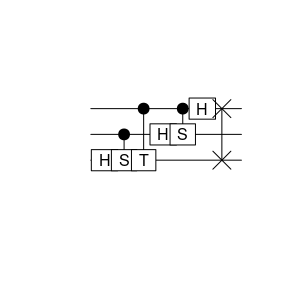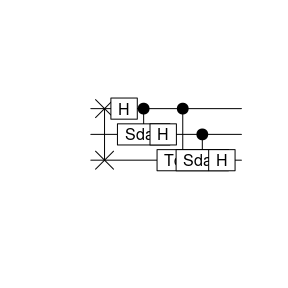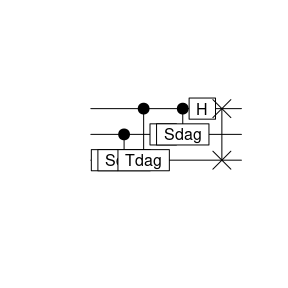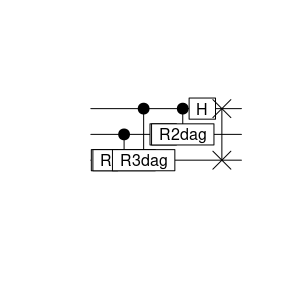# Three qubit QFT

Let’s take a normalised vector with eight components and Fourier transform it

N <- 3
v <- seq(1:2^N)
v <- v/sqrt(sum(v^2))

w <- fft(v, inverse=TRUE)/sqrt(length(v))

We have to use the Fast Fourier Trafo (FFT) here because R uses the convention where the phase is $$\exp\left(-2\pi i jk/n\right)$$ and the quantum Fourier Trafo uses the convention $$\exp\left(2\pi i jk/n\right)$$.

The same using the quantum Fourier Trafo (QFT). Note that we have to start with the most significant bit, which in this case is qubit 3.

x <- qstate(N, coefs=as.complex(v))
x <- H(3) * x
x <- cqgate(bits=c(2, 3), gate=S(3)) * x
x <- cqgate(bits=c(1, 3), gate=Tgate(3)) * x
x <- H(2) * x
x <- cqgate(bits=c(1, 2), gate=S(2)) * x
x <- H(1) * x
x <- SWAP(c(1,3)) * x

The corresponding circuit looks as follows

plot(x)Now the coefficients of the state x should be the Fourier transform of the coefficients of y. The QFT is unitary

sum(x@coefs*Conj(x@coefs))
 1+0i

and the coefficients match the one from the classical FFT

sqrt(sum((w - x@coefs)*Conj(w - x@coefs)))
 3.602883e-16+0i

# The inverse

Since the Hadamard gate is its own inverse, we need the hermitian conjugates for T and S

Tdagger <- function(bit) {
return(methods::new("sqgate",
bit=as.integer(bit),
M=array(as.complex(c(1., 0, 0, exp(-1i*pi/4))), dim=c(2,2)),
type="Tdag"))
}
Sdagger <- function(bit) {
return(methods::new("sqgate",
bit=as.integer(bit),
M=array(as.complex(c(1,0,0,-1i)), dim=c(2,2)),
type="Sdag"))
}

## Inverting the circuit

With these we can write the inverse QFT as follows, again for three qubits

z <- qstate(N, coefs=x@coefs)
z <- SWAP(c(1,3)) * z
z <- H(1) * z
z <- cqgate(bits=c(1, 2), gate=Sdagger(2)) * z
z <- H(2) * z
z <- cqgate(bits=c(1, 3), gate=Tdagger(2)) * z
z <- cqgate(bits=c(2, 3), gate=Sdagger(2)) * z
z <- H(3) * z
plot(z)The coefficients can be compared with the original vector we started from

sqrt(sum((v - z@coefs)*Conj(v - z@coefs)))
 2.058409e-16+0i

## Inverting the phase

Instead of inverting the circuit we can of course apply the usual inverse Fourier trafo, i.e. utilise the same circuit as for the original QFT with all phases reversed.

y <- qstate(N, coefs=x@coefs)
y <- H(3) * y
y <- cqgate(bits=c(2, 3), gate=Sdagger(3)) * y
y <- cqgate(bits=c(1, 3), gate=Tdagger(3)) * y
y <- H(2) * y
y <- cqgate(bits=c(1, 2), gate=Sdagger(2)) * y
y <- H(1) * y
y <- SWAP(c(1,3)) * y

plot(y)Again we get the vector v back we started with.

sqrt(sum((v - y@coefs)*Conj(v - y@coefs)))
 2.058409e-16+0i

## The general case

Let define a function performing a quanturm Fourier trafo for general number of qubits. For this we need a gate representing $R_k = \begin{pmatrix} 1 & 0 \\ 0 & \exp(2\pi \mathrm{i}/2^k) \\ \end{pmatrix}$ Such a general gate is easily implemented in qsimulatR as follows

Ri <- function(bit, i, sign=+1) {
type <- paste0("R", i)
if(sign < 0) {
type <- paste0("R", i, "dag")
}
return(methods::new("sqgate",
bit=as.integer(bit),
M=array(as.complex(c(1,0,0,exp(sign*2*pi*1i/2^i))),
dim=c(2,2)), type=type))
}

With this gate we can implement the quantum Fourier trafo function (the following function is almost identical to the qft function included in qsimulatR, see ?qft for details)

qft <- function(x, inverse=FALSE) {
n <- x@nbits
y <- x
sign <- +1
if(inverse) sign <- -1
for(bit in c(n:1)) {
y <- H(bit) * y
if(bit > 1) {
for(i in c((bit-1):1)) {
y <- cqgate(bits=c(i, bit), gate=Ri(bit, bit-(i-1), sign=sign)) * y
}
}
}
## reverse order
for(k in c(1:floor(n/2))) {
y <- SWAP(c(k, n-(k-1))) * y
}
return(invisible(y))
}

And we can plot and check the result again

y <- qstate(N, coefs=x@coefs)
y <- qft(y, inverse=TRUE)
plot(y)sqrt(sum((v - y@coefs)*Conj(v - y@coefs)))
 2.067318e-16+0i